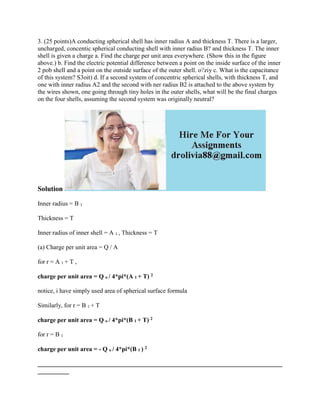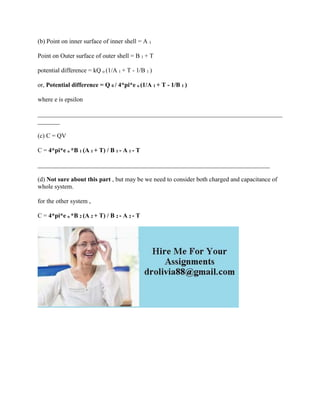Se está descargando tu SlideShare. ×

# 3- (25 points)A conducting spherical shell has inner radius A and thic.docx

Anuncio
Anuncio
Anuncio
Anuncio
Anuncio
Anuncio
Anuncio
Anuncio
Anuncio
Anuncio
AnuncioCargando en…3
×

1 de 2 Anuncio

# 3- (25 points)A conducting spherical shell has inner radius A and thic.docx

3. (25 points)A conducting spherical shell has inner radius A and thickness T. There is a larger, uncharged, concentic spherical conducting shell with inner radius B? and thickness T. The inner shell is given a charge a. Find the charge per unit area everywhere. (Show this in the figure above.) b. Find the electric potential difference between a point on the inside surface of the inner 2 pob shell and a point on the outside surface of the outer shell. o\'ziy c. What is the capacitance of this system? S3oit) d. If a second system of concentric spherical shells, with thickness T, and one with inner radius A2 and the second with ner radius B2 is attached to the above system by the wires shown, one going through tiny holes in the outer shells, what will be the final charges on the four shells, assuming the second system was originally neutral?
Solution
Thickness = T
Inner radius of inner shell = A 1 , Thickness = T
(a) Charge per unit area = Q / A
for r = A 1 + T ,
charge per unit area = Q o / 4*pi*(A 1 + T) 2
notice, i have simply used area of spherical surface formula
Similarly, for r = B 1 + T
charge per unit area = Q o / 4*pi*(B 1 + T) 2
for r = B 1
charge per unit area = - Q o / 4*pi*(B 1 ) 2
________________________________________________________________________________________
(b) Point on inner surface of inner shell = A 1
Point on Outer surface of outer shell = B 1 + T
potential difference = kQ o (1/A 1 + T - 1/B 1 )
or, Potential difference = Q 0 / 4*pi*e o (1/A 1 + T - 1/B 1 )
where e is epsilon
_____________________________________________________________________________________
(c) C = QV
C = 4*pi*e o *B 1 (A 1 + T) / B 1 - A 1 - T
__________________________________________________________________________
(d) Not sure about this part , but may be we need to consider both charged and capacitance of whole system.
for the other system ,
C = 4*pi*e o *B 2 (A 2 + T) / B 2 - A 2 - T
.

3. (25 points)A conducting spherical shell has inner radius A and thickness T. There is a larger, uncharged, concentic spherical conducting shell with inner radius B? and thickness T. The inner shell is given a charge a. Find the charge per unit area everywhere. (Show this in the figure above.) b. Find the electric potential difference between a point on the inside surface of the inner 2 pob shell and a point on the outside surface of the outer shell. o\'ziy c. What is the capacitance of this system? S3oit) d. If a second system of concentric spherical shells, with thickness T, and one with inner radius A2 and the second with ner radius B2 is attached to the above system by the wires shown, one going through tiny holes in the outer shells, what will be the final charges on the four shells, assuming the second system was originally neutral?
Solution
Thickness = T
Inner radius of inner shell = A 1 , Thickness = T
(a) Charge per unit area = Q / A
for r = A 1 + T ,
charge per unit area = Q o / 4*pi*(A 1 + T) 2
notice, i have simply used area of spherical surface formula
Similarly, for r = B 1 + T
charge per unit area = Q o / 4*pi*(B 1 + T) 2
for r = B 1
charge per unit area = - Q o / 4*pi*(B 1 ) 2
________________________________________________________________________________________
(b) Point on inner surface of inner shell = A 1
Point on Outer surface of outer shell = B 1 + T
potential difference = kQ o (1/A 1 + T - 1/B 1 )
or, Potential difference = Q 0 / 4*pi*e o (1/A 1 + T - 1/B 1 )
where e is epsilon
_____________________________________________________________________________________
(c) C = QV
C = 4*pi*e o *B 1 (A 1 + T) / B 1 - A 1 - T
__________________________________________________________________________
(d) Not sure about this part , but may be we need to consider both charged and capacitance of whole system.
for the other system ,
C = 4*pi*e o *B 2 (A 2 + T) / B 2 - A 2 - T
.

Anuncio
Anuncio

### 3- (25 points)A conducting spherical shell has inner radius A and thic.docx

1. 1. 3. (25 points)A conducting spherical shell has inner radius A and thickness T. There is a larger, uncharged, concentic spherical conducting shell with inner radius B? and thickness T. The inner shell is given a charge a. Find the charge per unit area everywhere. (Show this in the figure above.) b. Find the electric potential difference between a point on the inside surface of the inner 2 pob shell and a point on the outside surface of the outer shell. o'ziy c. What is the capacitance of this system? S3oit) d. If a second system of concentric spherical shells, with thickness T, and one with inner radius A2 and the second with ner radius B2 is attached to the above system by the wires shown, one going through tiny holes in the outer shells, what will be the final charges on the four shells, assuming the second system was originally neutral? Solution Inner radius = B 1 Thickness = T Inner radius of inner shell = A 1 , Thickness = T (a) Charge per unit area = Q / A for r = A 1 + T , charge per unit area = Q o / 4*pi*(A 1 + T) 2 notice, i have simply used area of spherical surface formula Similarly, for r = B 1 + T charge per unit area = Q o / 4*pi*(B 1 + T) 2 for r = B 1 charge per unit area = - Q o / 4*pi*(B 1 ) 2 ______________________________________________________________________________ __________
2. 2. (b) Point on inner surface of inner shell = A 1 Point on Outer surface of outer shell = B 1 + T potential difference = kQ o (1/A 1 + T - 1/B 1 ) or, Potential difference = Q 0 / 4*pi*e o (1/A 1 + T - 1/B 1 ) where e is epsilon ______________________________________________________________________________ _______ (c) C = QV C = 4*pi*e o *B 1 (A 1 + T) / B 1 - A 1 - T __________________________________________________________________________ (d) Not sure about this part , but may be we need to consider both charged and capacitance of whole system. for the other system , C = 4*pi*e o *B 2 (A 2 + T) / B 2 - A 2 - T